Core java - Interview Questions and Answers for 'Algor' | Search Interview Question - javasearch.buggybread.com# Search Interview QuestionsMore than 3000 questions in repository.There are more than 900 unanswered questions.Have a video suggestion.
Click Correct / Improve and please let us know.
Label / Company      Label / Company / TextSubmit Question

Core java - Interview Questions and Answers for 'Algor' - 5 question(s) found - Order By Newest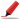Basic and Very Frequently asked.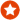Q1. What is Polymorphism in Java ? Core Java
Ans. Polymorphism means the condition of occurring in several different forms.

Polymorphism in Java is achieved in two manners

1. Static polymorphism is the polymorphic resolution identified at compile time and is achieved through function overloading whereas

2. Dynamic polymorphism is the polymorphic resolution identified at runtime and is achieved through method overriding.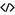Sample Code for overloadingSample Code for overridingHelp us improve. Please let us know the company, where you were asked this question :LikeDiscussCorrect / Improvepolymorphism  object oriented programming (oops)  oops concepts  oops concepts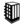Asked in 108 Companies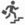Basic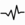frequent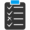Try 2 Question(s) TestQ2. Write a Program for Graph Depth First Traversal using Apache Commons MultiMap Algorithm
Ans. import java.util.ArrayList;
import java.util.Collection;
import java.util.HashSet;
import java.util.Set;

public class Graph {
private static Multimap<Integer,Integer> adjacentDirectedNodesMap = ArrayListMultimap.create();
private static Set<Integer> alreadyVisited = new HashSet();

static{
}

public static void main(String[] args){
ArrayList visited = new ArrayList();

Integer startNode = 1;

}

private static void displayAdjacentNodes(Integer integer){
return;
}
System.out.println(integer);
}
}

}Help us improve. Please let us know the company, where you were asked this question :LikeDiscussCorrect / Improvegraph traversal  depth first algorithmQ3. Which sorting algorithm is used by Collections.sort() in Java ? Core Java
Ans. The sorting algorithm is a modified mergesort. This algorithm offers guaranteed n log(n) performance.Help us improve. Please let us know the company, where you were asked this question :LikeDiscussCorrect / Improvejava   algorithm   collections   collections.sort   sorting algorithmexpertTry 1 Question(s) TestQ4. Write a Program for selection sort.
Ans. public class SelectionSort {
public static void main(String[] args){
int a[] = {1,3,4,5,7,8,2,6};

for(int i=0;i<a.length;i++){
for(int j=0;j<i;j++){
if(a[j] > a[j]){
int temporary = a[j];
a[j] = a[i];
a[i] = temporary;
}
}
}

for(int i=0;i<a.length;i++){
System.out.println(a[i]);
}
}
}Help us improve. Please let us know the company, where you were asked this question :LikeDiscussCorrect / Improvesorting algorithm  selection sortQ5. Write a program for bubble sort.
Ans. public class BubbleSort {
public static void main(String[] args){
int a[] = {1,3,4,5,7,8,2,6};

for(int i=0;i<a.length-1;i++){
for(int j=i;j<a.length;j++){
if(a[j] < a[j]){
int temporary = a[j];
a[j] = a[i];
a[i] = temporary;
}
}
}

for(int i=0;i<a.length;i++){
System.out.println(a[i]);
}
}
}Help us improve. Please let us know the company, where you were asked this question :LikeDiscussCorrect / Improvesorting algorithm  Bubble sortQ6. Binary Search requires that the collection should be Algorithm
a. Sorted in Ascending Order
b. Sorted in Descending Order
c. Sorted in any Order
d. Unsorted

Ans.c. Sorted in any Order

## Help us and Others Improve. Please let us know the questions asked in any of your previous interview.

Any input from you will be highly appreciated and It will unlock the application for 10 more requests.

 Company Name: Questions Asked: